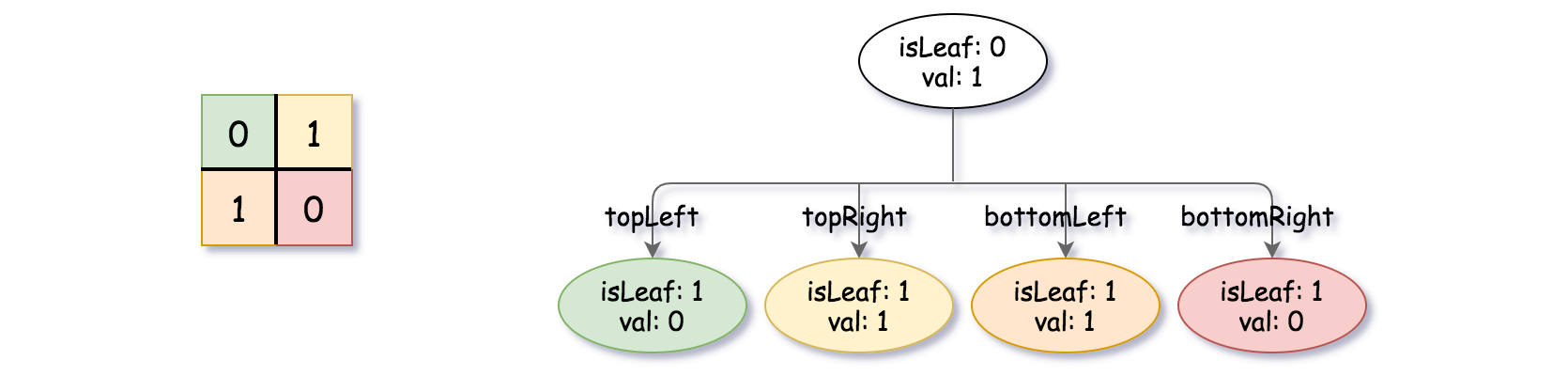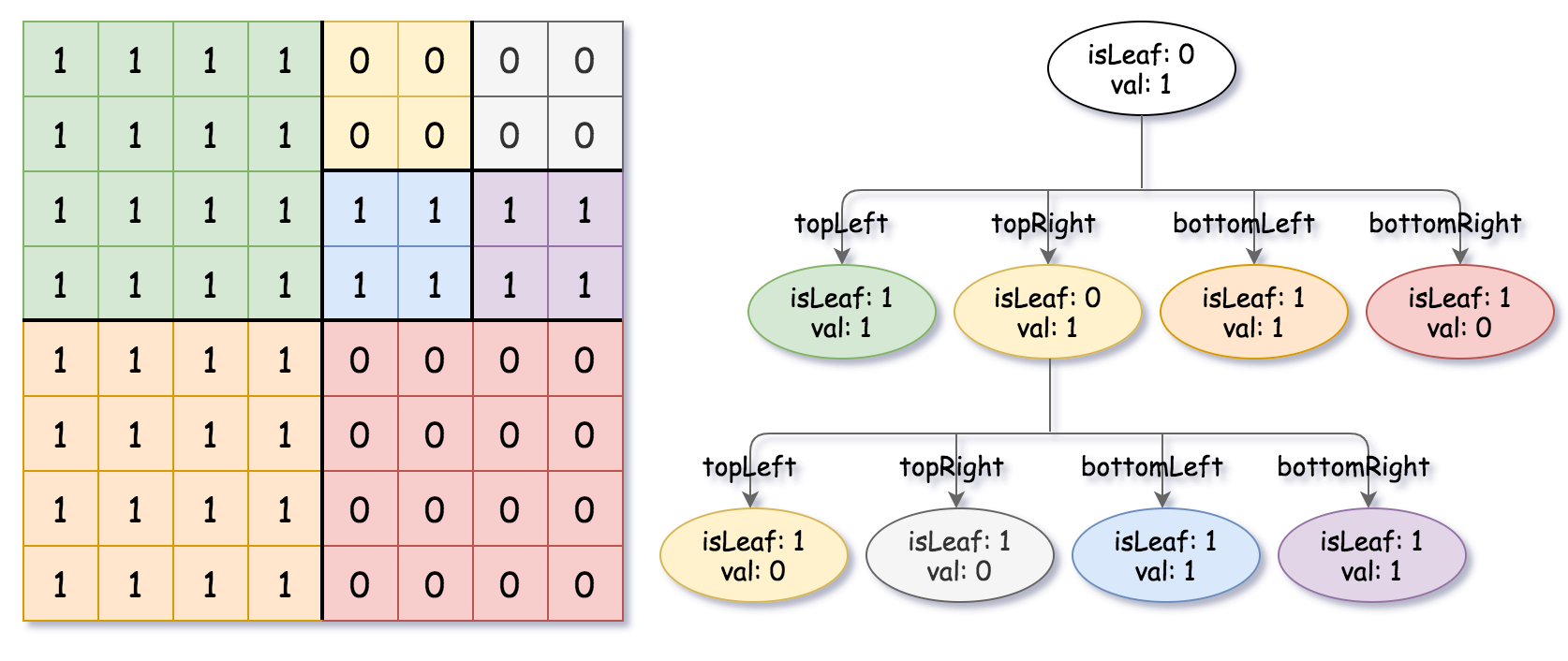# 427. Construct Quad Tree LeetCode Solution | Easy ApproachShare:

Construct Quad Tree Given a `n * n` matrix `grid` of `0's` and `1's` only. We want to represent the `grid` with a Quad-Tree.

Return the root of the Quad-Tree representing the `grid`.

Notice that you can assign the value of a node to True or False when `isLeaf` is False, and both are accepted in the answer.

A Quad-Tree is a tree data structure in which each internal node has exactly four children. Besides, each node has two attributes:

• `val`: True if the node represents a grid of 1’s or False if the node represents a grid of 0’s.
• `isLeaf`: True if the node is leaf node on the tree or False if the node has the four children.
```class Node {
public boolean val;
public boolean isLeaf;
public Node topLeft;
public Node topRight;
public Node bottomLeft;
public Node bottomRight;
}```

We can construct a Quad-Tree from a two-dimensional area using the following steps:

1. If the current grid has the same value (i.e all `1's` or all `0's`) set `isLeaf` True and set `val` to the value of the grid and set the four children to Null and stop.
2. If the current grid has different values, set `isLeaf` to False and set `val` to any value and divide the current grid into four sub-grids as shown in the photo.
3. Recurse for each of the children with the proper sub-grid.

If you want to know more about the Quad-Tree, you can refer to the wiki.

The output represents the serialized format of a Quad-Tree using level order traversal, where `null` signifies a path terminator where no node exists below.

It is very similar to the serialization of the binary tree. The only difference is that the node is represented as a list `[isLeaf, val]`.

If the value of `isLeaf` or `val` is True we represent it as 1 in the list `[isLeaf, val]` and if the value of `isLeaf` or `val` is False we represent it as 0.

Example 1:

```Input: grid = [[0,1],[1,0]]
Output: [[0,1],[1,0],[1,1],[1,1],[1,0]]
Explanation: The explanation of this example is shown below:
Notice that 0 represnts False and 1 represents True in the photo representing the Quad-Tree.```

Example 2:

```Input: grid = [[1,1,1,1,0,0,0,0],[1,1,1,1,0,0,0,0],[1,1,1,1,1,1,1,1],[1,1,1,1,1,1,1,1],[1,1,1,1,0,0,0,0],[1,1,1,1,0,0,0,0],[1,1,1,1,0,0,0,0],[1,1,1,1,0,0,0,0]]
Output: [[0,1],[1,1],[0,1],[1,1],[1,0],null,null,null,null,[1,0],[1,0],[1,1],[1,1]]
Explanation: All values in the grid are not the same. We divide the grid into four sub-grids.
The topLeft, bottomLeft and bottomRight each has the same value.
The topRight have different values so we divide it into 4 sub-grids where each has the same value.
Explanation is shown in the photo below:```

Constraints:

• `n == grid.length == grid[i].length`
• `n == 2x` where `0 <= x <= 6`

Time: O(n)
Space: O(n)

### C++

`` Will be updated Soon``

### Java

``````
Will be updated Soon``````

### Python

``````  Will be updated Soon
``````

#### Watch Tutorial

Checkout more Solutions here